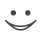Get these flashcards, study & pass exams. For free! Even on iPhone/Android!

Geometry (20 Cards)

If the three sides of one triangle are congruent to the three sides of another triangle, then the two triangles are congruent.
Side-Side-Side Postulate
The sum of the measures of of the angles of a triangle is 180.
Triangle Angle-Sum Theorem
The sum of the measures of the angles of an n-gon is (n-2) 180.
Polygon Exterior Angle-Sum Theorem
The sum of the lengths of any two sides of a triangle is greater than the length of the third side.
Triangle Inequality Theorem
If a segment joins the midpoint of two sides of a triangle, then the segment is parallel to the third side, and is half its length.
Triangle Midsegment Theorem
If a point is on the perpendicular bisector of a segment, then it is equdistant from the endpoints of the segment.
Perpendicular Bisector Theorem
If three points A,B and C are collinear and B is between A and C, then AB+BC=AC
If two lines and a transversal from corresponding angles that are congruent, then the two lines are parallel.
converse of the corresponding angles postulate
If a transversal intersects two parallel lines, then corresponding angles are congruent.
corresponding angles postulate
If two angles are complements of the same angle(or of congruent angles), then the two angles are congruent.
congruent complements theorem
If two sides of a triangle are congruent, then the angles opposite those sides are congruent.
Isosceles triangle theorem
If the hypotenuse and a leg of one right triangle are congruent to the hypotenuse and a leg of another right triangle, then the triangles are congruent.
Hypotenuse-Leg Theorem
If a conditional is true and its hypothesis occurs, its conclusion is guarenteed. In symbolic form: If p-->q is a true statement and p is true, then q is true.
Law of detachment
If a transversal intersects two parallel lines, then alternate interior angles are supplementary.
Same-side interior angles theorem
The measure of each exterior angle of a triangle equals the sum of the measure of its two remote interior angles.
Triangle exterior angle theorem
Vertical angles are congruent.
Verticle angles theorem
If a point is on the bisector of an angle, then the point is equidistant from the sides of the angle.
Angle Bisector Theorem
If p>q ad q>r are true statements, then p-->r is a true statement.
Law of Syllogism
Define CPCTC.
Corresponding Parts of Congruent Triangles are Congruent
Angles of a polygon that share a side.
Consecutive AnglesFlashcard set info:
Author: CoboCards-User
Main topic: Mathematics
Topic: Geometry
Published: 12.01.2011

Card tags:
All cards (20)
no tagsReport abuse